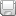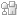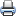# WDM Network DesignSaveDiggDel.icio.usPrint

### Chapter Description

This sample chapter explores some of the design constraints involved in the WDM network design.

## Examples

The following case studies reinforce the principles discussed so far in this chapter.

### Case 1

Design a 4 ×25 span WDM link with an optical amplifier gain of 22 dB and NF equal to 5 dB.

Calculate the final OSNR if the input power is 0 dB. Calculate the signal power at the receiver.

Will this system work if the receiver sensitivity is a minimum of –25 dB?

Will the system work if the input power is 10 dB?

The answer is shown in Figure 4-16.Figure 4-16 Answer to the Problem

You can use one of the two methods to determine the final OSNR.

#### Method 1#### Method 2

OSNR stage by stage analysis using the formula:

OSNRstagei = 1/(1/OSNRstage0 + NF.h.v.Δf /Pin) (1/OSNRstage0 = 0)

OSNR Stage 1

NF = 5 dB converting to linear = 3.166 (10NF dB/10)

h = Plank's constant = 6.6260E – 34

v = frequency of light 1.9350E + 14

Δf = bandwidth (measuring the NF) = 12.5 KHz (.1 nm)

Pin = input power at the amplifier

0 – 25 dB = –25 dB

OSNRstage1 = 28 dB

Output from the amplifier = –25 + 22 = –3 dB

OSNR Stage 2

OSNRstage2 = 1/(1/OSNRstage1 + NF.h.v.Δf /Pin)

Input at the second amplifier

= –3 – 25 = –28 dB

OSNRstage2 = 23 dB

Output from the amplifier = –28 + 22 = –6

OSNR Stage 3

OSNRstage3 =1/(1/OSNRstage3 + NF.h.v.Δf /Pin)

Input at the third amplifier = –6 – 25 = –31 dB

OSNRstage3 = 20 dB

Output from the amplifier = –31 + 22 = –9 dB

= –9 – 25 = –34 dB

If the receiver sensitivity is –25, the system will not work.

The solution is to (a) increase the gain of the amplifier (b) increase the input power of the transmitter.

The same solution for 10dB input power is shown in Figure 4-16.

Using Method 1: OSNRfinal = 10 + 58 – 25 – 6 – 5 = 32

Method 2: gives OSNRfinal = 29 dB

The difference in value is due to the approximation made in the parameters.

= 10(Tr) – 25 (loss1) + 22 (gain1) – 25 (loss2) + 22 (gain 2) – 25 (loss 3) + 22 (gain 3) – 25 (loss) = –24 dB

The receiver sensitivity is given as –25 dB, so the system will work.

### Case 2

Calculate the number of spans in this link, given Pin = 0 dB; OSNRfinal = 20 dB total length = 300 km Bit rate = 5 Gbps; NF = 5 dB. (Assume fiber type is SMF ∝ = .2 dB/km)

Total loss over entire length = 300 × 0.2 = 60 dB

Attenuation per span = 60/N (assume N number of spans

OSNRFinal = P0 + 58 ––10logN – NF

20 = 0 + 58 – 60/N –10logN – 5

-33 = –60/N – 10logN

Rearranging, you get this:

60/N – 10logN = 33

Solving for N (for N = 2)

60/2 + 10log2 = 33

Therefore, the number of spans = 2.

### Case 3

OSNR = 20 dB, dispersion of the fiber is 17 ps/nm-km, span loss = 22 dB.

What is the total length of the system? (NF of the amplifier = 4 dB, and dispersion tolerance is given as 1600 ps/nm, Pin = 10)

OSNRFinal = P0 + 58 ––10logN – NF

20 = 10 + 58 – 22 – 4 – 10logN

–22 = –10 logN

N = 158 spans

Therefore, total length = 158 * 22/0.2 = 17,280 km (theoretical limit)

But due to dispersion, max length = 1600 ps.nm/17 ps /nm.km = 94 km.

### Case 4

Customer A wants to build a 200 km OC48 link to transport traffic. Design the link with the following parameters. (Assume SMF-28 fiber with α = .25 dB/km/ and 18 ps/nm/km as the dispersion characteristic.)

Transmit power: +7 to +9 dBm

Dispersion tolerance: 1500 ps/nm

Dispersion penalty: 1.5 dB @ 1500 ps/nm

OSNR tolerance: 20 dB @ Resolution Bandwidth 0.1 nm

EDFA

Input power range: +3 to –25 dBm; Gain: 20 dB to 14 dB

Maximum output power: +17 dBm; Noise Figure: 5 dB

DCU

Loss 5 dB, dispersion compensation –1100 ps

The answer requires several steps. Refer to Figure 4-17.Figure 4-17 Case 4 Answer

 Step 1 The total distance is 200 km; therefore, total loss is 200 * .25 = 50 dB. You need amplifiers to reach that distances. Step 2 Total dispersion is 200 km * 18 ps.nm / km = 3600 ps.nm

You need to use dispersion compensators because the given dispersion tolerance is 1500 ps.nm. The maximum distance for this system without DCU is 1500/18 = 83.33 km given DCU = –1100 ps. If you strategically place 3 DCU, you get (3600 – 3 * 1100) = 300 ps, which is well within the limit. The DCU has a 5 dB passthrough loss, so it is best to place it before preamplifiers.

Analysis of the problem

Transmit power = + 7 dB

Stage 1:

loss = –10 (link loss) – 6 (DCU loss) – 1.5 (margin) = –17.5 dB

Pin = Power at the end of stage 1 = 7 – 17.5 = –10.5 dB

Stage 1 amplifier gain: 20

Power output from the amplifier: (power input + gain) – 10.5 dB + 20 = 9.5 dB

OSNR calculation

OSNRstagei =1/(1/OSNR0 + NF.h.v.Δf /Pin) (for stage 1 1/OSNR0 = 0)

OSNR1 = Pin/NF.h.v. Δf

Substituting the values Pin = –10.5 dB = 8.9125E – 05 W (convert to watts)

NF = 5 dB converting to linear = 3.166 (10NF dB/10)

h = planks constant = 6.6260E – 34

v = frequency of light 1.9350E + 14

Δf = bandwidth (measuring the NF) = 12.5 KHz (.1 nm)

Substituting, you get OSNR = 42 dB

Stage 2:

Loss = –20 (link loss – 6 (DCU loss) – 1.5 (margin) = –27.5 dB

Power at the end of stage 2

Pin = 9.5 – 20 – 6 – 1.5 (margin) = –18 dB

Stage 2 amplifier gain: 20

Power output from amplifier: –18 + 20 = 2 dB

OSNRstage2 = 1/(1/OSNR1 + NF.h.v.Δf /Pin)

OSNR1 is the OSNR of stage 1 = 42 dB and Pin = –18 dB

OSNRstage 2 = 34 dB

Stage 3 loss:

–20(link loss) – 6 (DCU loss) – 1.5 (margin) = –27.5 dB (loss)

Power at the end of stage 3

Pin= 2 – 27.5 = –25.5 dB

Stage 3 amplifier gain 20 dB

Power output from the amplifier: = –25.5 + 20 = –5.5 dB

OSNR

OSNRstage3 = 1/(1/OSNRstage2 + NF.h.v.Df /Pin)

OSNR = 26

### Case 5

Calculate the composite power at the output of a DWDM 8 channel multiplexor (shown in Figure 4-18) if the input power is 0 dB (insertion loss = 5 dB).Figure 4-18 Calculate the Composite Power

Pcomposite = Pchannel + 10logN – Insertion loss (where N is the number of channels)

= 0 + 10log8 – 5 dB = 0 + 9 – 5 dB

= 4 dB

### Exercises

The following exercises are left for you to solve. Use previous examples as guidelines.

1. Calculate the dispersion and the receiver power of an optical link that is 90 km in length. The transmit power = +8 dB, the receiver sensitivity = –18 dB, and the dispersion tolerance = 1500 ps/nm (SMF ∝ = .25 dB/km 18 ps/nm-km). Is it dispersion limited? Design the link for proper network operation if you need to use EDFA 15 dB (gain) and DCU –1100 ps and 5 dB loss).

2. Design a 4 × 25 span WDM link with optical amplifier gain = 18 dB and NF = 6 dB.

3. Calculate final OSNR if the input power is 0 dB. Calculate signal power at the receiver.

(The receiver sensitivity is –25 dB at BER 10-15). Does the system work?

Now calculate the final OSNR by replacing the following:

— The transmitter with input power to +7 dB

— The amplifier gain to 22 dB

4. Calculate power in milliwatts if the input power were 0 dBm.

5. Calculate the power in dBm if the input power were 12 mW.

Hint: XmW power is 10 * log10(x) in dBm

Y dBm power is 10Y/10 in mW

6. Calculate the composite power at the output of a DWDM 16-channel mux if the input power is 4 dB. If the input is plugged into an amplifier, how much attenuation do you need (given NF = 5 dB, input range = 0–25 dB, gain = 22)